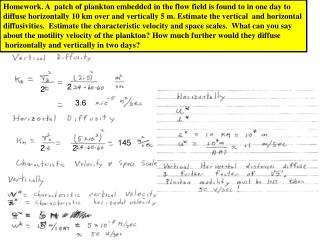DownloadDownload Presentation3.6

# 3.6

Download Presentation## 3.6

- - - - - - - - - - - - - - - - - - - - - - - - - - - E N D - - - - - - - - - - - - - - - - - - - - - - - - - - -
##### Presentation Transcript

1. Homework. A patch of plankton embedded in the flow field is found to in one day to diffuse horizontally 10 km over and vertically 5 m. Estimate the vertical and horizontal diffusivities. Estimate the characteristic velocity and space scales. What can you say about the motility velocity of the plankton? How much further would they diffuse horizontally and vertically in two days? 2 2 3.6 145 2 2

2. Homework: Suppose you release a large number of Lagrangian floats in a field of mesoscale eddies. You use a satellite tracking system tracking system which can only do one observation per day. If you know that these eddies are typically 50 km in horizontal extent with a decorrelation time of 10 days, how far would you expect the floats to diffuse in 100 days ? An SMAST student who has not yet taken physical oceanography tells you if you really want to impress your thesis adviser you should track these floats every day and every day tell your thesis adviser how far they have diffused. What do you think of that idea? (Answering that you do not want to bother your thesis adviser is not acceptable!) 1 day tracking not necessary and possibly misleading.

3. Homework: A patch of dye initially 5 m vertically by 100 m horizontally is located in a constant vertical velocity shear of . Microstructure turbulence measurements in this region suggest a vertical diffusivity of . How far does the patch disperse horizontally and vertically in 1 second, 1 minute, 1 hour, 1 day? When does shear dispersion effects become more important than the laminar stretching effects in determining horizontal structure.?

4. Homework: In a certain region on the continental shelf suppose there is a surface input of heat of F =200 Watts/m2. The turbulent diffusivity in this region is estimated as 10-4 m2/sec. If there is no upwelling what is the temperature gradient in the water. If there was an upwelling velocity of w = .1 mm/sec what would the temperature gradient profile be. What is the buoyancy profile?

5. Homework: The wind suddenly stops blowing and sets up inertial oscillations in the upper ocean. You place a float in the upper ocean and find that it moves around in a circle of radius 10 km. If you are located at 45 degrees N how long will it take the float to do a complete cycle? What is the speed which the floats move ? Suppose you deployed 10 such floats describe how each one would move relative to the others. They move together

6. Homework: Wind, , blows across the outer edge of the RI shelf to the east at 10 m/sec over a cross shelf extent of 50 km. CODAR measurements indicate a surface current magnitude of .1 m/sec, Assuming that the surface pressure gradient is very small, (a) What is the direction of the surface current? (b) What is the value of eddy viscosity?(c) What is the effective depth of the Ekman layer? (d) What is the magnitude and direction of the current field at 2 m below the surface, at 10 m below the surface? (e) What is your estimate of the turbulent velocity at the surface, at depths of 2, 10 meters, on the bottom ? (f) Estimate the upwelling velocity.

7. 2 2 2 Z=2m .057 35

8. .057 .057 45 Wind 45 28 East .29 35 (-.29) .75 .075) .75) .075 m/sec

9. -4

10. Homework: Along a west-east oriented continental shelf at latitude 42oN, of depth 100 meters, the sea surface has an cross shelf slope of 10-5, increasing to the south. In situ measurements from the T-REMUS vehicle indicate a near bottom eddy viscosity of k = 10-2 m2/sec. Assume that the wind is sufficiently light that it can be neglected. (a) What is the direction and magnitude of the surface current ; (b) what is the direction and magnitude of the bottom current? ( c) what is the “effective” thickness of the bottom Ekman layer? ( d) calculate the along shelf and cross shelf velocities at z = .5 m, 1m, 10 m off of the bottom.(e) What is the magnitude and direction of the bottom stress? (f) Estimate the magnitude of turbulent velocity at the bottom. (g) What is the direction of the near bottom mass transport anomaly (non geostrophic mass transport)?

11. Homework: At Station “A” wind blows to the north with a speed of . One degree due east of this location at Station B the wind blows to the north at . What is the Ekman pumping velocity w? Is it upward or downward?

12. Homework: Suppose for one year the average wind speed over the Atlantic Ocean is eastward and at 45 N while at 30 N (a) What is the magnitude and direction of the vertically integrated transport . (b) If we take the east-west extent of this region of the North Atlantic is 4000 km estimate the volume transport?